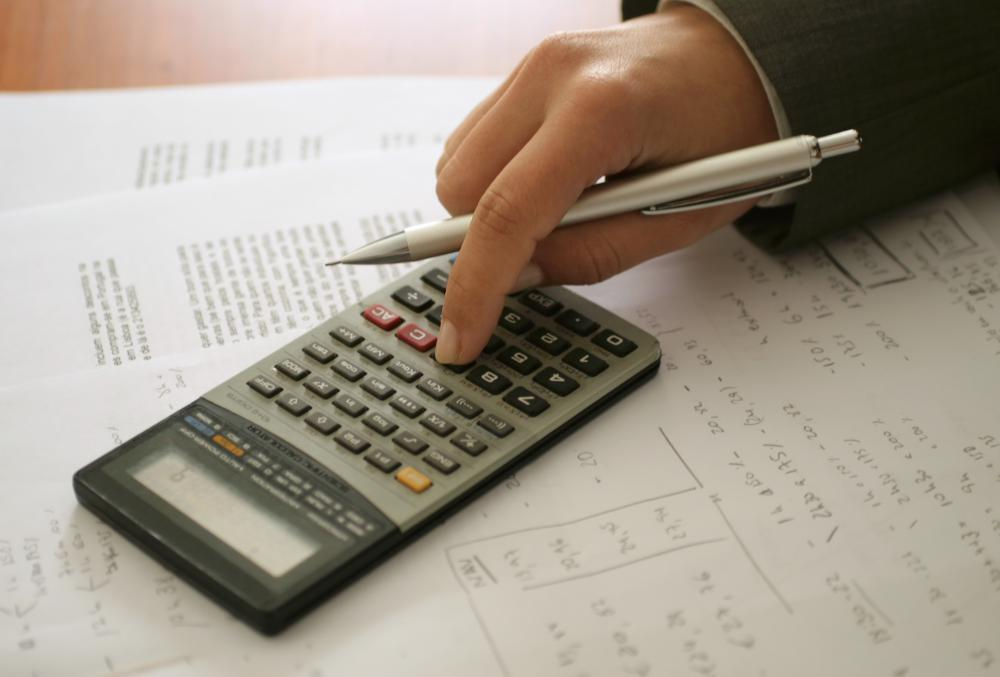# What are the Different Methods of Depreciation?

Jim B.

Depreciation refers to the decline in value that an asset incurs over the period of time in which it is used. This is a vital accounting concept because businesses write off the depreciation of their assets as an expense on tax returns, and there are several methods of depreciation used. The two most common methods of depreciation are straight-line depreciation, in which the value decreases the same amount each year, and declining balance depreciation, which calculates depreciation as a percentage of the balance of the asset's value. Other more complex methods include double-declining depreciation, which combines principles of straight-line and declining balance, activity depreciation, and the similar units-of-time methods.Declining balance depreciation calculates depreciation as a percentage of the balance of the asset's value.

Two important principles to understand no matter what methods of depreciation are used are depreciation expense and accumulated depreciation. The depreciation expense is the amount of depreciation value in one year's time. Accumulated depreciation is the total amount of depreciation the asset has undergone to that point. For example, a business vehicle depreciating in value at \$400 US Dollars (USD) per year would have an appreciation expense of \$400 USD each year on the business' balance sheet, but its accumulated depreciation would be \$400 USD in the first year, \$800 USD in the second, \$1200 USD in the third, and so on.

Straight-line depreciation is the simplest of the methods of depreciation and allows for the same amount of depreciation each year. For example, a computer costs \$1,000 USD and is to be used for five years. This means that it will be depreciating \$200 USD each year, which is reached by dividing \$1,000 USD by five.

Declining balance depreciation allows businesses to expense a purchase heaviest in the first year and then a declining amount each successive year. It accomplishes this by establishing a percentage rate of depreciation and applying that to the balance. Using the example above, if the computer's rate of depreciation was 50 percent, then it would depreciate \$500 USD the first year, or 50 percent of \$1,000 USD, leaving a balance of \$500 USD, or \$1,000 USD minus \$500 USD. In the next year, the depreciation expense would be \$250 USD, or 50 percent of the balance of \$500 USD, and that process would continue until the balance reached the salvage value of the computer.

The double declining method of depreciation uses the straight-line method to establish the percentage, double it, and then apply the doubled percentage rate to the declining method. Activity depreciation bases depreciation expenses on the amount an asset has been used as opposed to time, while the sum-of-years digits method is achieved by multiplying the original depreciable cost of the asset by a series of fractions based on the sum of the digits of the years the asset will be used. The units-of-production method is based on a formula that takes into account the amount of output produced by the asset, and the similar unit-of-time method applies this theory to natural resources that may become depleted over time.

## You might also Like